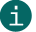# Check digit calculation of Modulo 43

Modulo 43

## This is a description of calculating check digits according to Modulo 43.ActiveBarcode calculates this check digits for most codes automatically.
This documentation is only for the sake of completeness.

Calculation of a checksum according to Modulo 43:
A check digit according to Modulo 43 is used e.g. by the Code 39.

First, reference numbers are assigned to all characters of the code. These reference numbers are added to a total. This sum is divided by 43. The rest of this division corresponds to the checksum, which is then replaced by the character corresponding to the reference number is represented.

A calculation example:

 Digits: 1 5 9 A Z Reference numbers: 1 +5 +9 +10 +35 Sum of reference numbers: 60 Calculate checksum: 60 / 43 = 1 Remainder 17 Check digit "H" (Reference number 17)

Reference numbers:
 00 0 01 1 02 2 03 3 04 4 05 5 06 6 07 7 08 8 09 9 10 A 11 B 12 C 13 D 14 E 15 F 16 G 17 H 18 I 19 J 20 K 21 L 22 M 23 N 24 O 25 P 26 Q 27 R 28 S 29 T 30 U 31 V 32 W 33 X 34 Y 35 Z 36 - 37 . 38 Space 39 \$ 40 / 41 + 42 %

## Code example for calculating the checksum according to Modulo 43

Excel VBA Code for calculating the checksum according to Modulo 43, e.g. for the HIBC code:
```' calc modulo 43
‘ returns the input string plus the check character
' demo for www.activebarcode.com
Public Function MOD43CheckChar(sValue As String) As String
Const charSet As String = "0123456789ABCDEFGHIJKLMNOPQRSTUVWXYZ-. \$/+%"
Dim i As Integer
Dim T As Long
For i = 1 To Len(Trim(UCase(sValue)))
T = InStr(charSet, Mid(sValue, i, 1)) - 1 + T
Next i
MOD43CheckChar = sValue & Mid\$(charSet, (T Mod 43 + 1), 1)
End Function
```